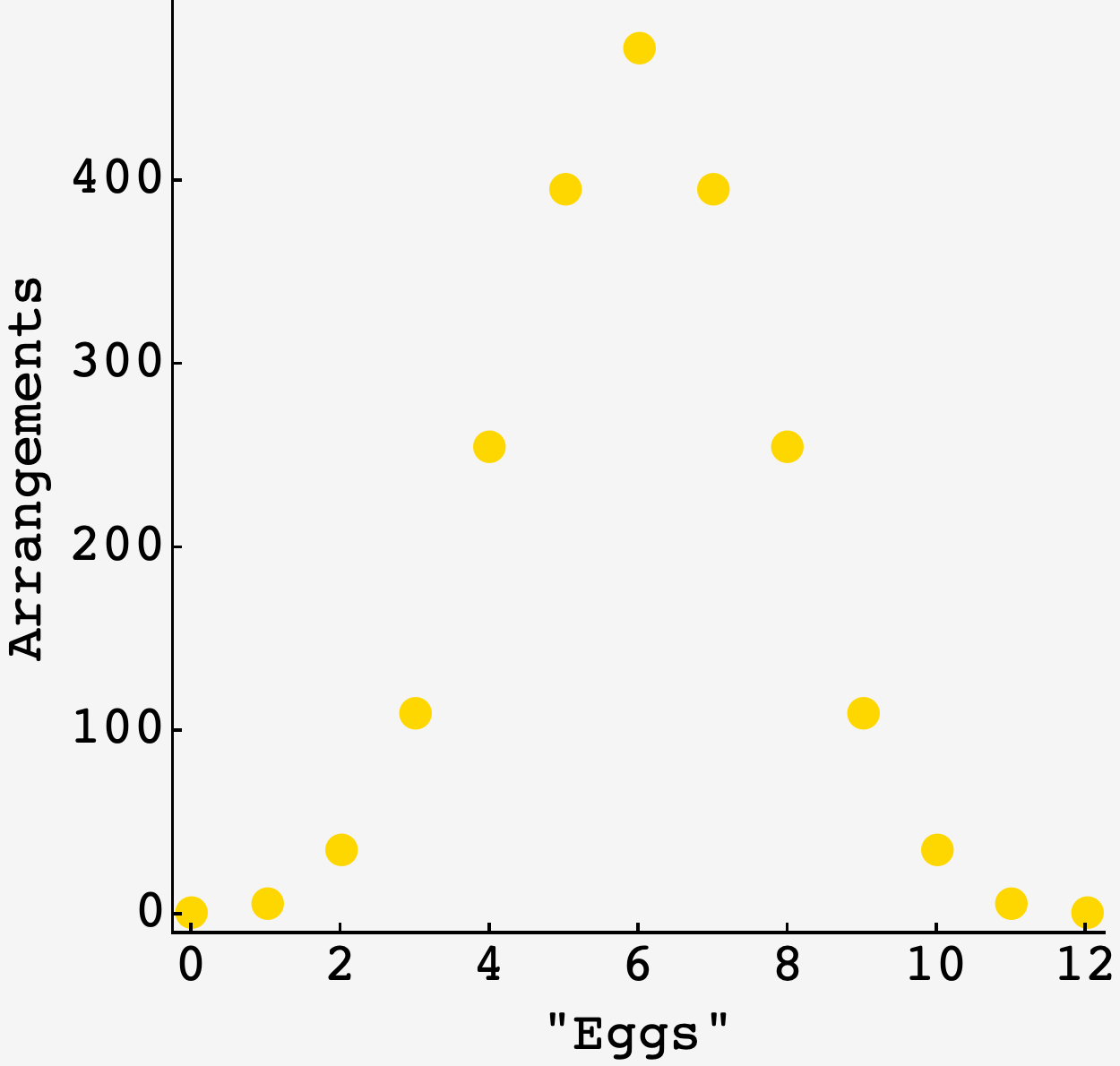Question: For Easter, you and your family decide to decorate $10$ beautiful eggs. You pull a fresh carton of eggs out of your fridge and remove $10$ eggs. There are two eggs remaining in the carton, which you return to the fridge.

The next day, you open the carton again to find that the positions of the eggs have somehow changed — or so you think. Perhaps the Easter Bunny was snooping around your fridge?

The $12$ slots in the carton are arranged in a six-by-two array that is symmetric upon a $180$-degree rotation, and the eggs are indistinguishable from each other. How many distinct ways are there to place two eggs in this carton? (Note: Putting two eggs in the two leftmost slots should be considered the same as putting them in the two rightmost slots, since you can switch between these arrangements with a 180-degree rotation of the carton.)

Extra credit: Instead of two eggs remaining, suppose you have other numbers of indistinguishable eggs between zero and $12.$ How many distinct ways are there to place these eggs in the carton?

## Solution

If we weren’t worried about rotational symmetry, then the answer would just be $\binom{12}{n},$ since that’s how many ways there are to place $n$ objects in $12$ receptacles.

But since we are worried about rotations, we need to make sure we count just one member from each each set of rotationally-equivalent arrangements.

The first thing to notice is that an arrangement with different numbers of eggs on either side of the middle can’t be rotationally equivalent to itself. This makes it straightforward to count cases with unequal numbers of eggs.

If there are $\ell$ eggs on the left, $r$ on the right, and $\ell \neq r,$ then there are $\binom{6}{\ell}\times\binom{6}{r}$ possible arrangements.

### Odd total number

So, for $5$ total eggs, the number of total arrangements is

$\Omega(5) = \binom{6}{5}\binom{6}{0} + \binom{6}{4}\binom{6}{1} + \binom{6}{3}\binom{6}{2} = 396.$

If we included terms with $\ell < r$ then we’d be counting the rotationally symmetric partners we want to exclude.

### Even total number

When there are an even total number of eggs, we have to include the $\ell = r$ case.

If we naively multiplied the number of arrangements for the left and right side of the carton, we would have a two-fold mess on our hands:

• There are $\binom{6}{\frac12n}$ cases where an arrangement pairs with its rotationally symmetric self. These contribute one arrangement to the total.
• Every other pairing has a rotationally symmetric duplicate represented in the product. These terms have to be downweighted by half to avoid double-counting.

This means that we have to separate these cases, downweight the second kind, and add them together. Carrying this out, the proper $\ell=r=\frac12 n$ term is

$\binom{6}{\frac12 n} + \frac12\left[\binom{6}{\frac12 n}^2 - \binom{6}{\frac12 n}\right] = \frac12\left[\binom{6}{\frac12 n} + \binom{6}{\frac12 n}^2\right].$

### Altogether

The last insight if that for $n \gt 6,$ the symmetry between holes and eggs means that $\Omega(n) = \Omega(12-n),$ and we don’t have to calculate them. Putting it all together, we get:

$\begin{array}{c|c} n & \Omega(n) \\ \hline 1 & 6 \\ 2 & 36 \\ 3 & 110 \\ 4 & 255 \\ 5 & 396 \\ 6 & 472 \\ \end{array}$

Plotting it: